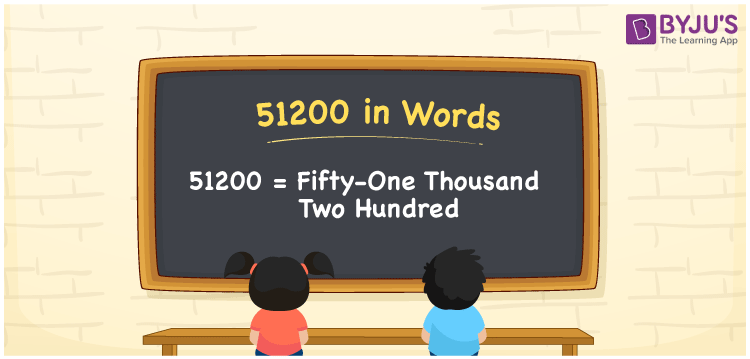# 51200 in Words

The number 51200 in words is Fifty-one Thousand Two Hundred. If you earned Rs. 51200 in 6 months, you can write it as “I earned Rs. Fifty-one thousand two hundred in six months”. Generally, we can convert any numbers into words using the place value system. Now, let us discuss how to write the spelling of 51200 in words with a complete explanation.

 51200 in Words: Fifty-one Thousand Two Hundred. Fifty-one Thousand Two Hundred in Numerical Form: 51200.

## 51200 in English Words## How to Write 51200 in Words?

The place value table for the number 51200 is provided below up to 5 digits.

 Ten-thousands Thousands Hundreds Tens Ones 5 1 2 0 0

The expanded form of 51200 is as follows:

= 5 × Ten thousand + 1 × Thousand + 2 × Hundred + 0 × Ten + 0 × One

= 5 × 10000 + 1 × 1000 + 2 × 100 + 0 × 10 + 0 ×1

= 50000 + 1000 + 200

= 51200

= Fifty-one thousand two hundred

Hence, 51200 in words is Fifty-one thousand two hundred.

51200 in words – Fifty-one thousand two hundred

Is 51200 an odd number? – No

Is 51200 an even number? – Yes

Is 51200 a perfect square number? – No

Is 51200 a perfect cube number? – No

Is 51200 a prime number? – No

Is 51200 a composite number? – Yes

## Frequently Asked Questions on 51200 in Words

Q1

### How to write 51200 in English words?

51200 in words is fifty-one thousand two hundred.

Q2

### Simplify 51000 + 200, and express it in words.

Simplifying 51000 + 200, we get 51200. Hence, 51200 in words is fifty-one thousand two hundred.

Q3

### Is 51200 an even number?

Yes, 51200 is an even number.